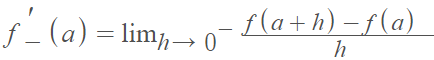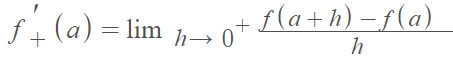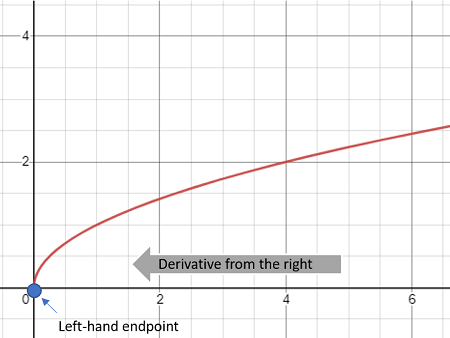# Left Hand Derivative & Right Hand Derivative

Sometimes we want to take the derivative at a point from one direction instead of both sides. When we approach a point from the left of the number line, it’s called a left hand derivative. When we approach from the right, it’s a right hand derivative. These definitions are exactly the same concept as one sided limits—limits from the left and limits from the right .

The definition of a left hand derivative at point a is:If the limit exists.

The definition of a right hand derivative at point a is:If the limit exists.

## Why use Right and Left Hand Derivative?

There are a few reasons why you might choose to use a left hand derivative or right hand derivative. The technique can be used to show that a derivative exists at a certain point: If both the left- and right-hand derivatives are equal, then the derivative at a exists.

Additionally, if you are finding the derivative for the endpoint in an interval, you have no choice but to use either a left- or right-hand derivative, because the function won’t be defined beyond those endpoints. For example, let’s say you wanted to find the derivative of √x. This function isn’t defined for values less than zero, so in order to find the derivative at x = 0, the left-hand endpoint, you need to use a right-hand derivative:The left-hand derivative clearly doesn’t exist (there’s nothing there to use for calculations!) and the right-hand derivative blows up to infinity; Therefore, as the left- and right-hand derivatives are not equal, the derivative at x = 0 does not exist.

## Example

Example question: Is the function f(x) = |x| + 1 differentiable at 0?

Solution: Find the left- and right-hand derivative.

The left hand derivative isThe right hand derivative isThese are not equal, so the derivative does not exist.

## References

 Reinholz, D. Derivatives. Retrieved August 10, 2021 from: https://www.ocf.berkeley.edu/~reinholz/ed/08sp_m160/lectures/derivatives.pdf

CITE THIS AS:
Stephanie Glen. "Left Hand Derivative & Right Hand Derivative" From StatisticsHowTo.com: Elementary Statistics for the rest of us! https://www.statisticshowto.com/left-hand-derivative-right-hand-derivative/